# Texas Go Math Grade 4 Lesson 2.5 Answer Key Relate Fractions, Decimals, and Money

Refer to our Texas Go Math Grade 4 Answer Key Pdf to score good marks in the exams. Test yourself by practicing the problems from Texas Go Math Grade 4 Lesson 2.5 Answer Key Relate Fractions, Decimals, and Money.

## Texas Go Math Grade 4 Lesson 2.5 Answer Key Relate Fractions, Decimals, and Money

Remember

1 dollar = 100 cents
1 quarter = 25 cents
1 dime = 10 cents
1 penny = 1 cent

Unlock the Problem

Together, Julie and Sarah have $1.00 in quarters. They want to share the quarters equally. How many quarters should each girl get? How much money is this? Use the model to relate money, fractions, and decimals.So, Each girl should get 2 quarters, or$ $$\frac{1}{2}$$.

Examples Use the money to model decimals.___________ cents
The given model is: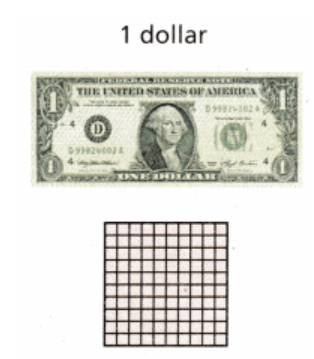Now,
We know that,
1 dollar = 100 cents
Hence, from the above,
We can conclude that
$1.00 = 100 centsSo,$ 0.10, or 10 centsSo,
$0.01, or 1 cent Relate Money and Decimals Think of dollars as ones, dimes as tenths, and pennies as hundredths.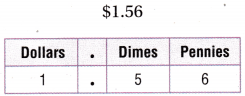Think:$1.56 = 1 dollar and 56 pennies
There are 100 pennies in 1 dollar
So,
$1.56 = 156 pennies.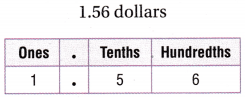Think: 1.56 = 1 one and 56 hundredths There are 100 hundredths in 1 one. So, 1.56 = 156 hundredths. Share and Show Question 1. Write the amount of money as a decimal in terms of dollars. 5 pennies = $$\frac{5}{100}$$ of a dollar = ___________ of a dollar.Answer: The given model is:Now, We know that, 1 dollar = 100 pennies So, 1 penny = $$\frac{1}{100}$$ dollar So, 5 pennies = 5 × $$\frac{1}{100}$$ = 5 × 0.01 = 0.05 dollars Hence, from the above, We can conclude that 5 pennies is equal to 0.05 of a dollar Write the total money amount. Then write the amount as a fraction or a mixed number and as a decimal in terms of dollars. Question 2.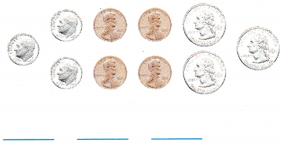Answer: The given model is: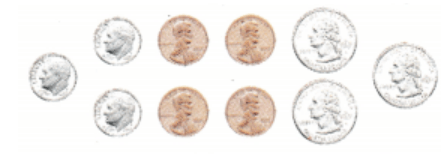Now, From the above model, We can observe that There are 4 pennies, 3 dimes, and 3 Quarters So, The total amount of money in dollars = (4 ×$0.01) + (3 × $0.1) + (3 ×$0.25)
= $0.04 +$0.3 + $0.75 =$1.09
Now,
The representation of the total amount of money in the form of a mixed number is: 1$$\frac{9}{100}$$
Hence, from the above,
We can conclude that
The representation of the total amount of money in the form of a mixed number is: 1$$\frac{9}{100}$$
The representation of the total amount of money in the form of a decimal number is: $1.09 Question 3.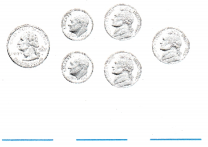Answer: The given model is:Now, From the above model, We can observe that There are 3 Quarters, 2 dimes, and 1 dollar So, The total amount of money in dollars = (3 ×$0.75) + (2 × $0.1) + (1 ×$1)
= $2.25 +$0.2 + $1 =$3.45
Now,
The representation of the total amount of money in the form of a mixed number is: 3$$\frac{45}{100}$$
Hence, from the above,
We can conclude that
The representation of the total amount of money in the form of a mixed number is: 3$$\frac{45}{100}$$
The representation of the total amount of money in the form of a decimal number is: $3.45 Write as a money amount and as a decimal in terms of dollars. Question 4. $$\frac{92}{100}$$ ________ ________ Answer: The given fraction is: $$\frac{92}{100}$$ Now, The representation of the given fraction in the form of a decimal is:$0.92
Hence, from the above,
We can conclude that
The representation of $$\frac{92}{100}$$ in the form of a decimal is: $0.92 Question 5. $$\frac{7}{100}$$ ________ ________ Answer: The given fraction is: $$\frac{7}{100}$$ Now, The representation of the given fraction in the form of a decimal is:$0.07
Hence, from the above,
We can conclude that
The representation of $$\frac{7}{100}$$ in the form of a decimal is: $0.07 Question 6. $$\frac{16}{100}$$ ________ ________ Answer: The given fraction is: $$\frac{16}{100}$$ Now, The representation of the given fraction in the form of a decimal is:$0.16
Hence, from the above,
We can conclude that
The representation of $$\frac{16}{100}$$ in the form of a decimal is: $0.16 Question 7. $$\frac{53}{100}$$ ________ ________ Answer: The given fraction is: $$\frac{53}{100}$$ Now, The representation of the given fraction in the form of a decimal is:$0.53
Hence, from the above,
We can conclude that
The representation of $$\frac{53}{100}$$ in the form of a decimal is: $0.53 Math Talk Mathematical Processes Explain how$0.84 and $$\frac{84}{100}$$ a dollar are related.
The given decimal number is: $0.84 Now, We know that, The number of digits after the decimal point represents the number of zeroes in the denominator after 1 i.e., 10 (or) 100 So, The representation of$0.84 in the form of a fraction is: $$\frac{84}{100}$$
Hence, from the above,
We can conclude that
The representation of $0.84 in the form of a fraction is: $$\frac{84}{100}$$ Problem Solving H.O.T. Algebra Complete to tell the value of each digit. Question 8.$1.05 = ________ dollar + ________ pennies, 1.05 = ________ ones + ________ hundredths
The given number is: $1.05 Now, We know that, We will consider dollars as “Ones”, dimes as “Tenths”, and pennies as “Hundredths” So,$1.05 = 1 dollar + 0 dimes + 5 pennies
= 1 one + 5 hundredths
Hence, from the above,
We can conclude that
$1.05 = 1 dollar + 5 pennies 1.05 = 1 ones + 5 hundredths Question 9.$5.18 = ________ dollars + ________dime + ________ pennies
5.18 = ________ ones + ________ tenth + ________ hundredths
The given number is: $5.18 Now, We know that, We will consider dollars as “Ones”, dimes as “Tenths”, and pennies as “Hundredths” So,$5.18 = 5 dollars + 1 dime + 8 pennies
= 5 ones + 1 tenth +  8 hundredths
Hence, from the above,
We can conclude that
$5.18 = 5 dollars + 1dime + 8 pennies 5.18 = 5 ones + 1 tenth + 8 hundredths Question 10. Sam has 3 dollar bills, 2 quarters, 3 dimes, and 2 pennies in her pocket. When this amount of money is written in terms of dollars, what is the value of the digit in the tenth place? hundredths place? Answer: It is given that Sam has 3 dollar bills, 2 quarters, 3 dimes, and 2 pennies in her pocket So, The total amount of money = (3 ×$1) + (2 × $0.25) + (3 ×$0.1) + (2 × $0.01) =$3 + $0.50 +$0.3 + $0.02 =$3.82
Now,
The representation of 3.82 in the place-value chart is: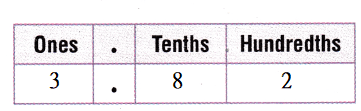Hence, from the above,
We can conclude that
The value of the digit in the tenths place is: 0.8
The value of the digit in the hundredths place is: 0.02

Use the table for 11-12.Question 11.
Use Diagrams The table shows the coins three students have. Write Nick’s total amount as a decimal and as a fraction in terms of dollars.
It is given that
The table shows the coins three students have
Now,
The given table is:Now,
From the above table,
We can observe that
Nock has 2 Quarters, 4 dimes, 0 Nickels, and 2 pennies
So,
The total amount of money Nick has = ( 2 × $0.25) + (4 ×$0.1) + (2 × $0.01) =$0.50 + $0.4 +$0.02
= $0.92 So, The representation of 0.92 in the form of a fraction is: $$\frac{92}{100}$$ Hence, from the above, We can conclude that The representation of the total amount of money Nick has in the form of a fraction is: $$\frac{92}{100}$$ The representation of the total amount of money Nick has in the form of a decimal number is:$0.92

Question 12.
H.O.T. Kim spent $$\frac{40}{100}$$ of a dollar on a snack. Write as a money amount the amount she has left.
It is given that
Kim spent $$\frac{40}{100}$$ of a dollar on a snack
Now,
The given table is:Now,
From the above table,
We can observe that
Kim has 1 Quarter, 3 dimes, 2 Nickels, and 3 pennies
So,
The total amount of money Kim has = (1 × $0.25) + (3 ×$0.1) + (2 × $0.05) + (3 ×$0.01)
= $0.25 +$0.3 + $0.1 +$0.03
= $0.68 = $$\frac{68}{100}$$ So, The amount of money Kim has left = $$\frac{68}{100}$$ – $$\frac{40}{100}$$ = $$\frac{68 – 40}{100}$$ = $$\frac{28}{100}$$ Hence, from the above, We can conclude that The amount of money Kim has left is: $$\frac{28}{100}$$ of a dollar Question 13. Jane has 2 dimes, 6 nickels, and 10 pennies in her pocket. What fraction of a dollar does Jane have in her pocket? Answer: It is given that Jane has 2 dimes, 6 nickels, and 10 pennies in her pocket Now, According to the given information, The fraction of a dollar Jane has in her pocket = (2 ×$0.1) + (6 × $0.05) + (10 ×$0.01)
= $0.2 +$0.3 + $0.1 =$0.6
= $$\frac{6}{10}$$
= $$\frac{60}{100}$$ of a dollar
Hence, from the above,
We can conclude that
The fraction of a dollar Jane has in her pocket is: $$\frac{60}{100}$$ of a dollar

Question 14.
H.O.T. Multi-Step Travis has $$\frac{50}{100}$$ of a dollar. He has at least two different types of coins in his pocket. Draw two possible sets of coins that Travis could have.
It is given that
Travis has $$\frac{50}{100}$$ of a dollar. He has at least two different types of coins in his pocket.
Now,
The representation of $$\frac{50}{100}$$ in the form of a decimal number is: $0.50 Now, According to the given information,$0.50 = (3 × $0.1) + (4 ×$0.05)
= 3 dimes + 4 nickels
Now,
$0.50 = 2 ×$0.25
= 2 Quarters
Hence, from the above,
We can conclude that
The two possible sets of coins that Travis could have is:
a. 3 dimes and 4 nickels
b. 2 Quarters

Question 15.
The price written on a puzzle at a garage sale is $$\frac{85}{100}$$. What is this amount written as a decimal in terms of dollars?
(A) 0.85
(B) 8.05
(C) 8.5
(D) 85.00
It is given that
The price written on a puzzle at a garage sale is $$\frac{85}{100}$$.
Now,
The representation of $$\frac{85}{100}$$ in the form of a decimal is: 0.85
Hence, from the above,
We can conclude that
The representation of $$\frac{85}{100}$$ in the form of a decimal is: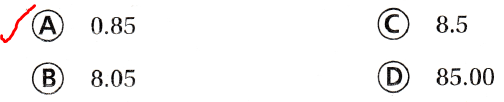Question 16.
The coins below show how much money Debbie gave her brother.What is the amount written as a decimal in terms of dollars?
(A) 0.70
(B) 0.27
(C) 0.72
(D) 7.2
The given money is:Now,
From the above,
We can observe that
The amount of money Debbie gave her brother is: 7 dimes, and 2 pennies
So,
The total amount of money Debbie gave her brother = (7 × $0.1) + (2 ×$0.01)
= $0.7 +$0.02
= $0.72 Hence, from the above, We can conclude that The total amount of money Debbie gave her brother is:Question 17. David has$0.68 left after buying lunch at school. Which shows the money amount written as a fraction in terms of dollars?
(A) $$\frac{68}{10}$$
(B) $$\frac{8}{10}$$
(C) $$\frac{6}{100}$$
(D) $$\frac{68}{100}$$
It is given that
David has $0.68 left after buying lunch at school Now, The representation of$0.68 in the form of a fraction = $$\frac{68}{100}$$
Hence, from the above,
We can conclude that
The representation of $0.68 in the form of a fraction is: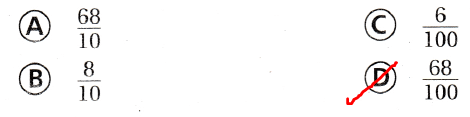Texas Test Prep Question 18. Mia has two dollars and fifteen cents. What decimal names this money amount in terms of dollars? (A) 2.50 (B) 2.15 (C) 21.50 (D) 0.15 Answer: It is given that Mia has two dollars and fifteen cents Now, We know that, 1 dollar = 100 cents So, The total amount of money Mia has = (2 × 100) + 15 = 200 + 15 = 215 cents = $$\frac{215}{100}$$ of a dollar =$2.15
Hence, from the above,
We can conclude that
The total amount of money Mia has is: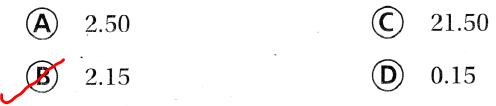### Texas Go Math Grade 4 Lesson 2.5 Homework and Practice Answer Key

Complete to tell the value of each digit.

Question 1.
$4.38 = ________ dollars + ________ dimes + ________ pennies$4.38 = ________ ones + ________ tenths + ________ hundredths
The given number is: $4.38 Now, We know that, We will consider dollars as “Ones”, dimes as “Tenths”, and pennies as “Hundredths” So,$4.38 = 4 dollars + 3 dimes + 8 pennies
= 4 ones + 3 tenths + 8 hundredths
Hence, from the above,
We can conclude that
$4.38 = 4 dollars + 3 dimes + 8 pennies$4.38 = 4 ones + 3 tenths + 8 hundredths

Question 2.
$2.05 = ________ dollars + ________ dimes + ________ pennies$2.05 = ________ ones + ________ tenths + ________ hundredths
The given number is: $2.05 Now, We know that, We will consider dollars as “Ones”, dimes as “Tenths”, and pennies as “Hundredths” So,$2.05 = 2 dollars + 0 dimes + 5 pennies
= 2 ones + 0 tenths + 5 hundredths
Hence, from the above,
We can conclude that
$2.05 = 2 dollars + 0 dimes + 5 pennies$2.05 = _2 ones + 0 tenths + _5 hundredths

Write as a money amount and as a decimal in terms of dollars.

Question 3.
$$\frac{74}{100}$$ _________ _________
The given fraction is: $$\frac{74}{100}$$
Now,
The representation of the given fraction in the form of a decimal is: $0.74 Hence, from the above, We can conclude that The representation of $$\frac{74}{100}$$ in the form of a decimal is:$0.74

Question 4.
$$\frac{31}{100}$$ _________ _________
The given fraction is: $$\frac{31}{100}$$
Now,
The representation of the given fraction in the form of a decimal is: $0.31 Hence, from the above, We can conclude that The representation of $$\frac{31}{100}$$ in the form of a decimal is:$0.31

Question 5.
$$\frac{69}{100}$$ _________ _________
The given fraction is: $$\frac{69}{100}$$
Now,
The representation of the given fraction in the form of a decimal is: $0.69 Hence, from the above, We can conclude that The representation of $$\frac{69}{100}$$ in the form of a decimal is:$0.69

Question 6.
$$\frac{58}{100}$$ _________ _________
The given fraction is: $$\frac{58}{100}$$
Now,
The representation of the given fraction in the form of a decimal is: $0.58 Hence, from the above, We can conclude that The representation of $$\frac{58}{100}$$ in the form of a decimal is:$0.58

Question 7.
$$\frac{83}{100}$$ _________ _________
The given fraction is: $$\frac{83}{100}$$
Now,
The representation of the given fraction in the form of a decimal is: $0.83 Hence, from the above, We can conclude that The representation of $$\frac{83}{100}$$ in the form of a decimal is:$0.83

Question 8.
$$\frac{95}{100}$$ _________ _________
The given fraction is: $$\frac{95}{100}$$
Now,
The representation of the given fraction in the form of a decimal is: $0.95 Hence, from the above, We can conclude that The representation of $$\frac{95}{100}$$ in the form of a decimal is:$0.95

Question 9.
$$\frac{12}{100}$$ _________ _________
The given fraction is: $$\frac{12}{100}$$
Now,
The representation of the given fraction in the form of a decimal is: $0.12 Hence, from the above, We can conclude that The representation of $$\frac{12}{100}$$ in the form of a decimal is:$0.12

Question 10.
$$\frac{26}{100}$$ _________ _________
The given fraction is: $$\frac{26}{100}$$
Now,
The representation of the given fraction in the form of a decimal is: $0.26 Hence, from the above, We can conclude that The representation of $$\frac{26}{100}$$ in the form of a decimal is:$0.26

Problem Solving

Use the table for 11-13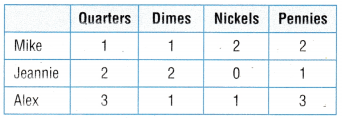Question 11.
The table tells the coins three people have. Write Mike’s total amount as a decimal and as a fraction in terms of a dollar.
It is given that
The table tells the coins three people have
Now,
The given table is:Now,
From the above table,
We can observe that
Mike has 1 Quarter, 1 Dime, 2 Nickels, and 2 pennies
So,
The total amount of money Mike has = (1 × $0.25) + (1 ×$0.1) + (2 × $0.05) + (2 ×$0.01)
= $0.25 +$0.1 + $0.1 +$0.02
= $0.47 Now, The representation of 0.47 in the form of a fraction is: $$\frac{47}{100}$$ Hence, from the above, We can conclude that The representation of the total amount of money Mike has in the form of a fraction is: $$\frac{47}{100}$$ of a dollar The representation of the total amount of money Mike has in the form of a decimal is:$0.47

Question 12.
Write Jeannie’s total amount. Then write the amount as a fraction of a dollar and as a decimal in terms of dollars.
The given table is:Now,
From the above table,
We can observe that
Jeannie has 2 Quarters, 2 Dimes, 0 Nickels, and 1 penny
So,
The total amount of money Mike has = (2 × $0.25) + (2 ×$0.1) + (0 × $0.05) + (1 ×$0.01)
= $0.5 +$0.2 + $0 +$0.01
= $0.71 Now, The representation of 0.71 in the form of a fraction is: $$\frac{71}{100}$$ Hence, from the above, We can conclude that The representation of the total amount of money Jeannie has in the form of a fraction is: $$\frac{71}{100}$$ of a dollar The representation of the total amount of money Jeannie has in the form of a decimal is:$0.71

Question 13.
If Alex spends $$\frac{63}{100}$$ of a dollar, how much money will he have left?
It is given that
Alex spends $$\frac{63}{100}$$ of a dollar
Now,
The given table is:Now,
From the above table,
We can observe that
Alex has 3 Quarters, 1 Dime, 1 Nickel, and 3 Pennies
So,
The total amount of money Alex has = (3 × $0.25) + (1 ×$0.1) + (1 × $0.05) + (3 ×$0.01)
= $0.75 +$0.1 + $0.05 +$0.03
= $0.93 Now, The representation of 0.93 in the form of a fraction is: $$\frac{93}{100}$$ So, The amount of money Alex has left = $$\frac{93}{100}$$ – $$\frac{63}{100}$$ = $$\frac{93 – 63}{100}$$ = $$\frac{30}{100}$$ of a dollar =$0.30
Hence, from the above,
We can conclude that
The amount of money Alex has left is: $$\frac{30}{100}$$ of a dollar

Lesson Check

Question 14.
Which shows $0.87 written as a fraction in terms of dollars? (A) 8$$\frac{7}{10}$$ (B) 8$$\frac{7}{100}$$ (C) $$\frac{87}{10}$$ (D) $$\frac{87}{100}$$ Answer: The given number is:$0.87
Now,
The representation of the given decimal in the form of a fraction is: $$\frac{87}{100}$$
Hence, from the above,
We can conclude that
The representation of $0.87 in the form of a fraction is:Question 15. Write the total value of these coins as a decimal in terms of dollars.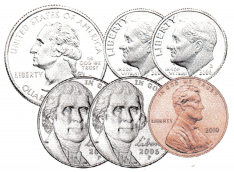(A) 0.56 (B) 0.056 (C) 5.06 (D) 56 Answer: The given coins are:Now, From the above coins, We can observe that The total value of the given coins = (1 ×$0.25) + (1 × $0.01) + (2 ×$0.05) + (2 × $0.1) =$0.25 + $0.01 +$0.1 + $0.2 =$0.56
Hence, from the above,
We can conclude that
The total value of the given coins is: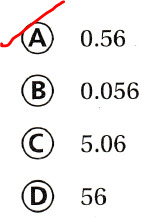Question 16.
Roxanna has $$\frac{42}{100}$$ of a dollar in change. Which shows the amount of change she has?
(A) $4.20 (B)$42
(C) $0.42 (D)$1.00
It is given that
Roxanna has $$\frac{42}{100}$$ of a dollar in change
Now,
The representation of $$\frac{42}{100}$$ as a decimal is: 0.42
Hence, from the above,
We can conclude that
The amount of change Roxanna has is:Question 17.
Jimmy has $$\frac{30}{100}$$ of a dollar. Which shows this amount in decimal form?
(A) 0.33
(B) 1.03
(C) 1.3
(D) 0.3
It is given that
Jimmy has $$\frac{30}{100}$$ of a dollar
Now,
The representation of $$\frac{30}{100}$$ as a decimal is: 0.30
Hence, from the above,
We can conclude that
The representation of $$\frac{30}{100}$$ as a decimal is: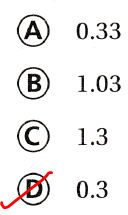Question 18.
Multi-Step Jesse has 3 quarters and 4 dimes. If he spends $$\frac{1}{2}$$ of a dollar, how much money will he have left?
(A) $0.65 (B)$0.50
(C) $0.55 (D)$0.60
It is given that
Jesse has 3 quarters and 4 dimes and he spends $$\frac{1}{2}$$ of a dollar
Now,
The representation of $$\frac{1}{2}$$ of a dollar in the decimal form is: $0.5 The total amount of money Jesse has = (3 ×$0.25) + (4 × $0.1) =$0.75 + $0.4 =$1.15
Now,
The amount of money Jesse has left = $1.15 –$0.5
= $0.65 Hence, from the above, We can conclude that The amount of money Jesse has left is:Question 19. Multi-Step Jenny has 4 dollar hills, 2 quarters, 1 dime, and 3 pennies. When this amount of money is written as a decimal in terms of dollars, which digit is in tenths place? (A) 3 (B) 6 (C) 4 (D) 5 Answer: It is given that Jenny has 4 dollar hills, 2 quarters, 1 dime, and 3 pennies Now, The total amount of money Jenny has = (4 ×$1) + (2 × $0.25) + (1 ×$0.1) + (3 × $0.01) =$4 + $0.5 +$0.1 + $0.03 =$4.63
Now,
The representation of 4.63 in the place-value chart is: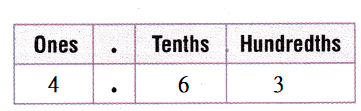Hence, from the above,
We can conclude that
The digit that is present in tenths place in the total amount of Jenny has is: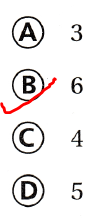Scroll to Top